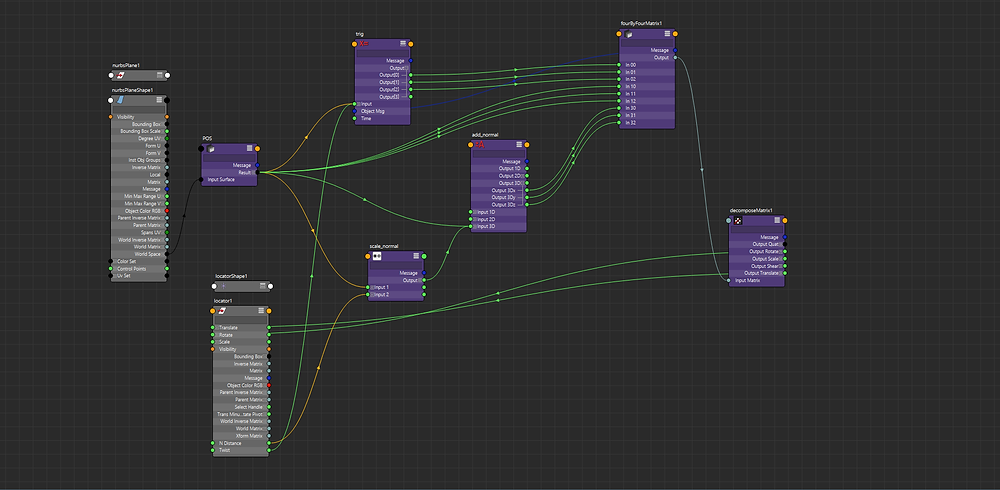# Custom Rivet

So I have a pretty good understanding of how matrices work in Maya, but that's a very different thing from being able to apply that knowledge. So I decided to try and create a matrix based rivet.

So attaching a locator to a surface is easy if you know about the four-by-four matrix node. Just create a point on surface info node and plug the position, normal vector, and one of the tangent vectors into the matrix and then turn the matrix into tranform attributes using a decompose matrix node.

http://bindpose.com/maya-matrix-nodes-part-3-matrix-rivet/

But that's been done before, so I wanted to try for something more complicated. I wanted to implement a twist and normal distance attribute on the rivet. The end result was this.The difference between this system and a simple matrix rivet constraint is that instead of directly plugging in the position, I scale the normal vector and add it to the position vector. I also do some trigonometry with the normal vector and the surface tangent vector to create the twist (that's what the expression node is there for).

In retrospect using a compose matrix node and a multiply matrix node would have been a far easier way to implement twist, but it was nice to practice applying trig to Maya (and refresh my expression syntax knowledge).

If I ever decide to revamp my surface based facial rigging script then this would be a much better method of turning vectors into transformations (originally I used aim constraints).

This was also great practice for understanding the math behind the UVN deformer using matrices.